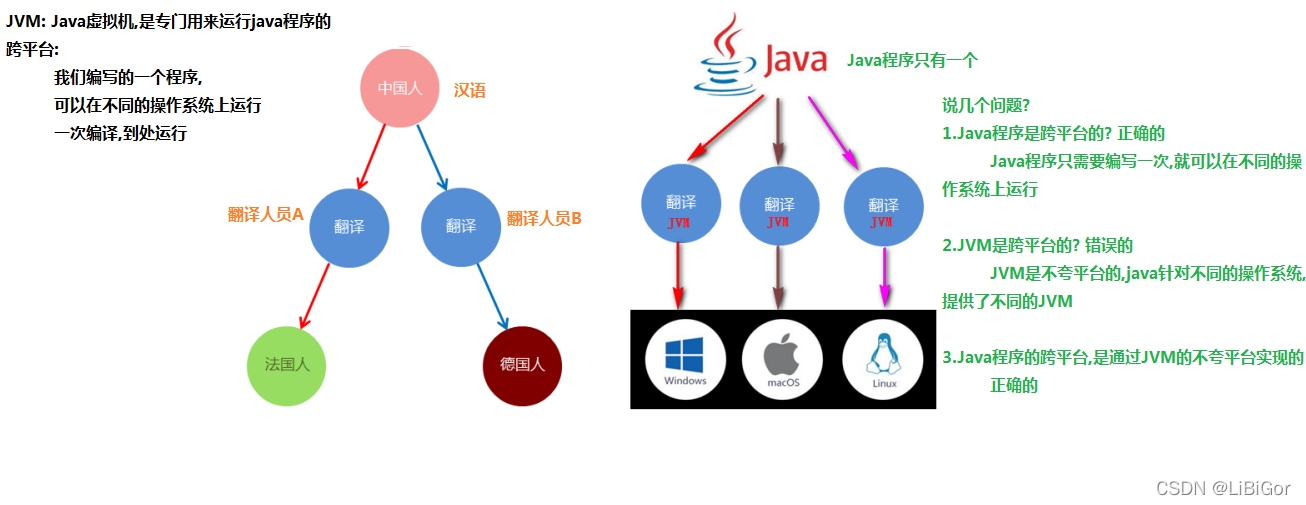# Java随机算法（一）(r11笔记第14天)

+关注继续查看

http://blog.sina.com.cn/blog_4f925fc30100uvur.html

(数据类型)(最小值+Math.random()*(最大值-最小值+1))

(int)(1+Math.random()*(10-1+1))

for (int i=0;i<30;i++)
{System.out.println((int)(1+Math.random()*10));}
(int)(1+Math.random()*10)

（类型）最小值+Math.random()*最大值

Random ra =new Random();
for (int i=0;i<30;i++)
{System.out.println(ra.nextInt(10)+1);}

[n1，n2]

java产生随机数的几种方式

java.util.Random类有两种方式构建方式：带种子和不带种子

public class RandomTest {
public static void main(String[] args) {
java.util.Random r=new java.util.Random();
for(int i=0;i<10;i++){

System.out.println(r.nextInt());
}

}

public static void main(String[] args) {
java.util.Random r=new java.util.Random(10);
for(int i=0;i<10;i++){

System.out.println(r.nextInt());
}
}

(1) 首先请打开Java Doc，我们会看到Random类的说明：

Java Doc对Random类已经解释得非常明白，我们的测试也验证了这一点。

(2) 如果没有提供种子数，Random实例的种子数将是当前时间的毫秒数，可以通过System.currentTimeMillis()来获得当前时间的毫秒数。打开JDK的源代码，我们可以非常明确地看到这一点。
public Random() { this(System.currentTimeMillis()); }

random对象的nextInt(),nextInt(int n)方法的说明：

int nextInt()

返回下一个伪随机数，它是此随机数生成器的序列中均匀分布的 int 值。
int nextInt(int n)

返回一个伪随机数，它是从此随机数生成器的序列中取出的、在 0（包括）和指定值（不包括）之间均匀分布的int值。

# Java随机数总结

随机数在实际中使用很广泛，比如要随即生成一个固定长度的字符串、数字。或者随即生成一个不定长度的数字、或者进行一个模拟的随机选择等等。Java提供了最基本的工具，可以帮助开发者来实现这一切。

一、Java随机数的产生方式

在Java中，随机数的概念从广义上将，有三种。

1、通过System.currentTimeMillis()来获取一个当前时间毫秒数的long型数字。

2、通过Math.random()返回一个0到1之间的double值。

3、通过Random类来产生一个随机数，这个是专业的Random工具类，功能强大。

二、Random类API说明

1、Java API说明

Random类的实例用于生成伪随机数流。此类使用 48 位的种子，使用线性同余公式对其进行修改(请参阅 Donald Knuth 的《The Art of Computer Programming， Volume 2》，第 3.2.1 节)。

如果用相同的种子创建两个 Random 实例，则对每个实例进行相同的方法调用序列，它们将生成并返回相同的数字序列。为了保证属性的实现，为类 Random 指定了特定的算法。

很多应用程序会发现 Math 类中的 random 方法更易于使用。

2、方法摘要

Random()

创建一个新的随机数生成器。

Random(long seed)

使用单个 long 种子创建一个新随机数生成器： public Random(long seed) { setSeed(seed); } next 方法使用它来保存随机数生成器的状态。

protected int next(int bits)

生成下一个伪随机数。

boolean nextBoolean()

返回下一个伪随机数，它是从此随机数生成器的序列中取出的、均匀分布的 boolean 值。

void nextBytes(byte[] bytes)

生成随机字节并将其置于用户提供的字节数组中。

double nextDouble()

返回下一个伪随机数，它是从此随机数生成器的序列中取出的、在 0.0 和 1.0之间均匀分布的 double 值。

float nextFloat()

返回下一个伪随机数，它是从此随机数生成器的序列中取出的、在 0.0 和 1.0 之间均匀分布的 float 值。

double nextGaussian()

返回下一个伪随机数，它是从此随机数生成器的序列中取出的、呈高斯(“正常地”)分布的 double 值，其平均值是 0.0，标准偏差是 1.0。

int nextInt()

返回下一个伪随机数，它是此随机数生成器的序列中均匀分布的 int 值。

int nextInt(int n)

返回一个伪随机数，它是从此随机数生成器的序列中取出的、在 0(包括)和指定值(不包括)之间均匀分布的 int值。

long nextLong()

返回下一个伪随机数，它是从此随机数生成器的序列中取出的、均匀分布的 long 值。

void setSeed(long seed)

使用单个 long 种子设置此随机数生成器的种子。

三、Random类使用说明

1、带种子与不带种子的区别Random类使用的根本是策略分带种子和不带种子的Random的实例。

通俗说，两者的区别是：带种子的，每次运行生成的结果都是一样的。

不带种子的，每次运行生成的都是随机的，没有规律可言。

2、创建不带种子的Random对象

Random random = new Random();

3、创建不带种子的Random对象有两种方法：

1) Random random = new Random(555L);

2) Random random = new Random();random.setSeed(555L);

四、测试

通过一个例子说明上面的用法

import java.util.Random;

public class TestRandomNum {

public static void main(String[] args) {

randomTest();

testNoSeed();

testSeed1();

testSeed2();

}

public static void randomTest() {

System.out.println("--------------test()--------------");

//返回以毫秒为单位的当前时间。

long r1 = System.currentTimeMillis();

//返回带正号的 double 值，大于或等于 0.0，小于 1.0。

double r2 = Math.random();

//通过Random类来获取下一个随机的整数

int r3 = new Random().nextInt();

System.out.println("r1 = " + r1);

System.out.println("r3 = " + r2);

System.out.println("r2 = " + r3);

}

public static void testNoSeed() {

System.out.println("--------------testNoSeed()--------------");

//创建不带种子的测试Random对象

Random random = new Random();

for (int i = 0; i < 3; i++) {

System.out.println(random.nextInt());

}

}

public static void testSeed1() {

System.out.println("--------------testSeed1()--------------");

//创建带种子的测试Random对象

Random random = new Random(555L);

for (int i = 0; i < 3; i++) {

System.out.println(random.nextInt());

}

}

public static void testSeed2() {

System.out.println("--------------testSeed2()--------------");

//创建带种子的测试Random对象

Random random = new Random();

random.setSeed(555L);

for (int i = 0; i < 3; i++) {

System.out.println(random.nextInt());

}

}

}

运行结果：

--------------test()--------------

r1 = 1227108626582

r3 = 0.5324887850155043

r2 = -368083737

--------------testNoSeed()--------------

809503475

1585541532

-645134204

--------------testSeed1()--------------

-1367481220

292886146

-1462441651

--------------testSeed2()--------------

-1367481220

292886146

-1462441651

Process finished with exit code 0

通过testSeed1()与testSeed2()方法的结果可以看到，两个打印结果相同，因为他们种子相同，再运行一次，结果还是一样的，这就是带种子随机数的特性。

而不带种子的，每次运行结果都是随机的。

五、综合应用

下面通过最近写的一个随机数工具类来展示用法：

import java.util.Random;

public class RandomUtils {

public static final String allChar = "0123456789abcdefghijklmnopqrstuvwxyzABCDEFGHIJKLMNOPQRSTUVWXYZ";

public static final String letterChar = "abcdefghijklmnopqrstuvwxyzABCDEFGHIJKLMNOPQRSTUVWXYZ";

public static final String numberChar = "0123456789";

public static String generateString(int length) {

StringBuffer sb = new StringBuffer();

Random random = new Random();

for (int i = 0; i < length; i++) {

sb.append(allChar.charAt(random.nextInt(allChar.length())));

}

return sb.toString();

}

public static String generateMixString(int length) {

StringBuffer sb = new StringBuffer();

Random random = new Random();

for (int i = 0; i < length; i++) {

sb.append(allChar.charAt(random.nextInt(letterChar.length())));

}

return sb.toString();

}

public static String generateLowerString(int length) {

return generateMixString(length).toLowerCase();

}

public static String generateUpperString(int length) {

return generateMixString(length).toUpperCase();

}

public static String generateZeroString(int length) {

StringBuffer sb = new StringBuffer();

for (int i = 0; i < length; i++) {

sb.append('0');

}

return sb.toString();

}

public static String toFixdLengthString(long num, int fixdlenth) {

StringBuffer sb = new StringBuffer();

String strNum = String.valueOf(num);

if (fixdlenth - strNum.length() >= 0) {

sb.append(generateZeroString(fixdlenth - strNum.length()));

} else {

throw new RuntimeException("将数字" + num + "转化为长度为" + fixdlenth + "的字符串发生异常!");

}

sb.append(strNum);

return sb.toString();

}

public static String toFixdLengthString(int num, int fixdlenth) {

StringBuffer sb = new StringBuffer();

String strNum = String.valueOf(num);

if (fixdlenth - strNum.length() >= 0) {

sb.append(generateZeroString(fixdlenth - strNum.length()));

} else {

throw new RuntimeException("将数字" + num + "转化为长度为" + fixdlenth + "的字符串发生异常!");

}

sb.append(strNum);

return sb.toString();

}

public static void main(String[] args) {

System.out.println(generateString(15));

System.out.println(generateMixString(15));

System.out.println(generateLowerString(15));

System.out.println(generateUpperString(15));

System.out.println(generateZeroString(15));

System.out.println(toFixdLengthString(123, 15));

System.out.println(toFixdLengthString(123L, 15));

}

}

运行结果：

vWMBPiNbzfGCpHG

23hyraHdJkKPwMv

tigowetbwkm1nde

BPZ1KNEJPHB115N

000000000000000

000000000000123

000000000000123

Process finished with exit code 0

六、总结

1、随机数很常用，在Java有三种产生方式，以Random随机数的使用最为复杂。

2、Random类对象有是否带种子之分，带种子的只要种子相同，多次运行，生成随机数的结果总是那样。

3、带种子随机数的带种子的对象创建方式有两种，效果一样。但是带种子的随机数用处似乎不大。

4、Random的功能涵盖了Math.random()的功能。

5、可以通过随机数去做实现随机字符串等复杂的随机数据。

6、不要研究不重复的随机数，意义不大。

随机数发生器(Random)对象产生以后，通过调用不同的method：nextInt()、nextLong()、nextFloat()、nextDouble()等获得不同类型随机数。

1>生成随机数

Random random = new Random();

Random random = new Random(100);//指定种子数100

random调用不同的方法，获得随机数。

如果2个Random对象使用相同的种子（比如都是100），并且以相同的顺序调用相同的函数，那它们返回值完全相同。如下面代码中两个Random对象的输出完全相同

import java.util.*;

class TestRandom {

public static void main(String[] args) {

Random random1 = new Random(100);

System.out.println(random1.nextInt());

System.out.println(random1.nextFloat());

System.out.println(random1.nextBoolean());

Random random2 = new Random(100);

System.out.println(random2.nextInt());

System.out.println(random2.nextFloat());

System.out.println(random2.nextBoolean());

}

}

2>指定范围内的随机数

随机数控制在某个范围内,使用模数运算符%

import java.util.*;

class TestRandom {

public static void main(String[] args) {

Random random = new Random();

for(int i = 0; i < 10;i++) {

System.out.println(Math.abs(random.nextInt()));

}

}

}

获得的随机数有正有负的，用Math.abs使获取数据范围为非负数

3>获取指定范围内的不重复随机数

import java.util.*;

class TestRandom {

public static void main(String[] args) {

int[] intRet = new int;

int intRd = 0; //存放随机数

int count = 0; //记录生成的随机数个数

int flag = 0; //是否已经生成过标志

while(count<6){

Random rdm = new Random(System.currentTimeMillis());

intRd = Math.abs(rdm.nextInt())2+1;

for(int i=0;i<count;i++){

if(intRet[i]==intRd){

flag = 1;

break;

}else{

flag = 0;

}

}

if(flag==0){

intRet[count] = intRd;

count++;

}

}

for(int t=0;t<6;t++){

System.out.println(t+"->"+intRet[t]);

}

}

}
Java中的随机数是否可以重复？Java中产生的随机数能否可以用来产生数据库主键？带着这个问题，我们做了一系列测试。
1.测试一： 使用不带参数的Random()构造函数

public class RandomTest {

public static void main(String[] args) {

java.util.Random r=new java.util.Random();

for(int i=0;i<10;i++){

System.out.println(r.nextInt());

}
}
}

-1761145445
-1070533012
216216989
-910884656
-1408725314
-1091802870
1681403823
-1099867456
347034376
-1277853157

-169416241
220377062
-1140589550
-1364404766
-1088116756
2134626361
-546049728
1132916742
-1522319721
1787867608

从上面的测试我们可以看出，使用不带参数的Random()构造函数产生的随机数不会重复。那么，什么情况下Java会产生重复的随机数呢？且看下面的测试。

2. 测试二：为Random设置种子数
public class RandomTest_Repeat {

public static void main(String[] args) {

java.util.Random r=new java.util.Random(10);

for(int i=0;i<10;i++){

System.out.println(r.nextInt());

}

}
}

-1157793070
1913984760
1107254586
1773446580
254270492
-1408064384
1048475594
1581279777
-778209333
1532292428

通过上面的测试和分析，我们会对Random类有较为深刻的理解。同时，我觉得，通过阅读Java Doc的API文档，可以很好地提高我们的Java编程能力，做到“知其然”；一旦遇到费解的问题，不妨打开Java的源代码，这样我们就能做到“知其所以然”。Java版点餐小程序2021最新版笔记，springboot+Mysql+freemarker+微信小程序实现扫码点餐小程序（上）
Java版点餐小程序2021最新版笔记，springboot+Mysql+freemarker+微信小程序实现扫码点餐小程序
36 0Java_基础阶段笔记总结汇总
JVM: Java虚拟机，是专门用来运行Java程序的,但是不能单独安装
27 027 058 029 084 042 0Java架构速成笔记：七大专题，1425页考点，挑战P8岗

53 050 058 0
jeanron100
Oracle ACE，《Oracle DBA工作笔记》作者 现就职于国内某互联网公司，擅长数据管理，数据迁移，性能优化，目前专注于开源技术，运维自动化和性能优化。

Java编程入门1497630

JAVA开发手册1.5.0